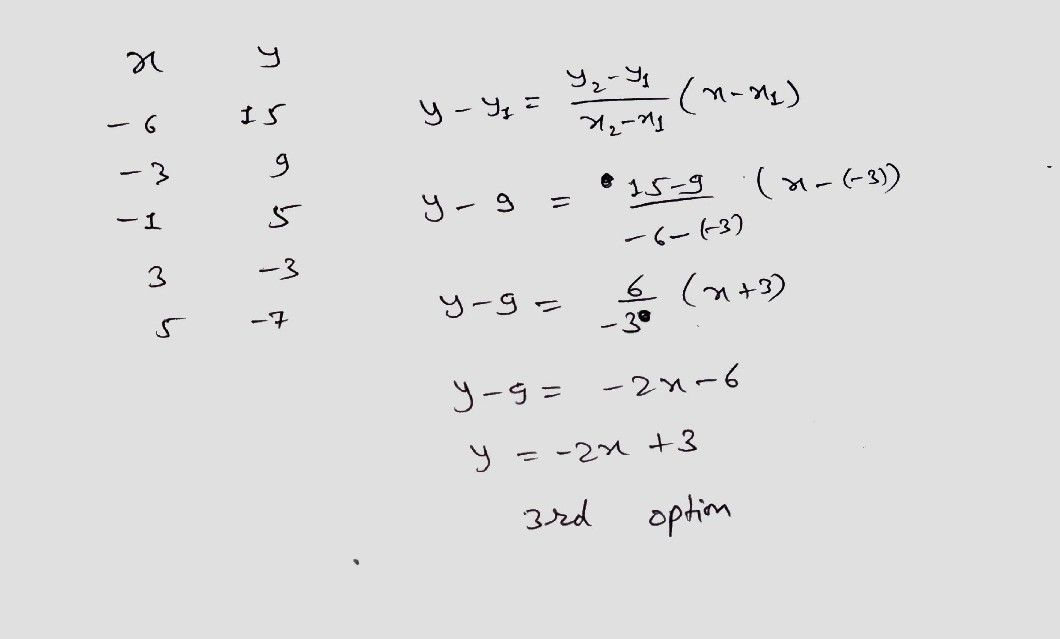Symbol
Problem$x$ $y$ $-6$ $15$ $-3$ $9$ Write a linear equation in the $-1$ $5$ $3$ $-3$ form of $\square$ $\right)230$ $5$ $-7$ $=243s5$ $1=323=4$ $=2$ $3$ $=223$